# quadratic function into standard form

y = -x2 + 4x + 11
(a) Express the quadratic in standard form.
Find any axis intercepts.
(x, y) =
(smallest x-value)

(x, y) =
(largest x-value)

This Homework Help Question: "quadratic function into standard form" No answers yet.

We need 10 more requests to produce the answer to this homework help question. Share with your friends to get the answer faster!

0 /10 have requested the answer to this homework help question.

Once 10 people have made a request, the answer to this question will be available in 1-2 days.
All students who have requested the answer will be notified once they are available.

#### Earn Coin

Coins can be redeemed for fabulous gifts.

Similar Homework Help Questions
• ### graph the quadratic function. Find the x- and y-intercepts of each graph, if any exist. If...

graph the quadratic function. Find the x- and y-intercepts of each graph, if any exist. If it is given in general form, convert it into standard form; if it is given in standard form, convert it into general form. Find the domain and range of the function and list the intervals on which the function is increasing or decreasing. Identify the vertex and the axis of symmetry and determine whether the vertex yields a relative and absolute maximum or minimum....

• ### 17) Given the quadratic function g(x)= -x2 - 4x + 4 a) Express the quadratic function...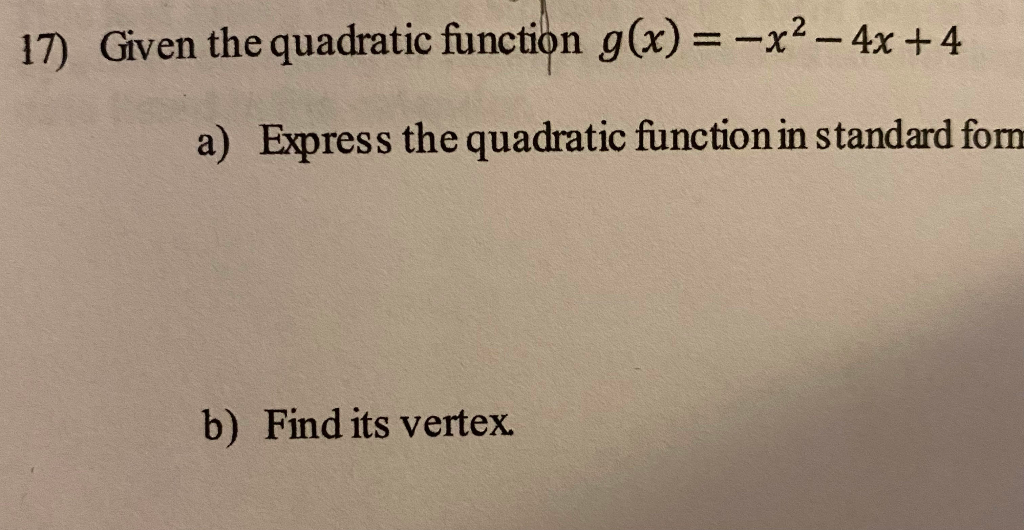17) Given the quadratic function g(x)= -x2 - 4x + 4 a) Express the quadratic function in standard forn b) Find its vertex.

• ### A quadratic function f is given. flx) = -x2. 4x 3 (a) Express f in transformation...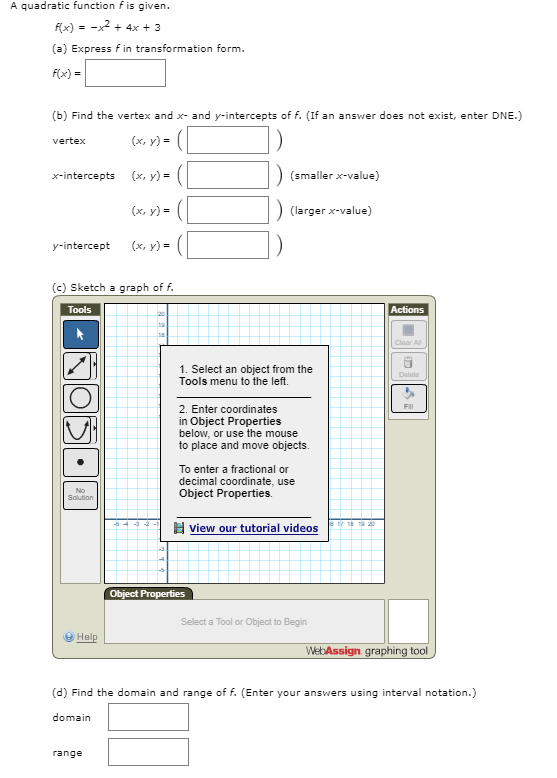A quadratic function f is given. flx) = -x2. 4x 3 (a) Express f in transformation form. f(x) (b) Find the vertex and x and y-intercepts of f. (If an answer does not exist, enter DNE.) (x, y) vertex (x, y) (smaller x-value) x-intercepts (x, y) (larger x-value) (x, y) yintercept (c) Sketch a graph of f. Tools Actions Clear Al 1. Select an object from the Tools menu to the left. Delato 2. Enter coordinates in Object Properties below,...

• ### + 6/7 points Previous Answers ColAlg7 3.1.009. A quadratic function f is given. f(x) = x2...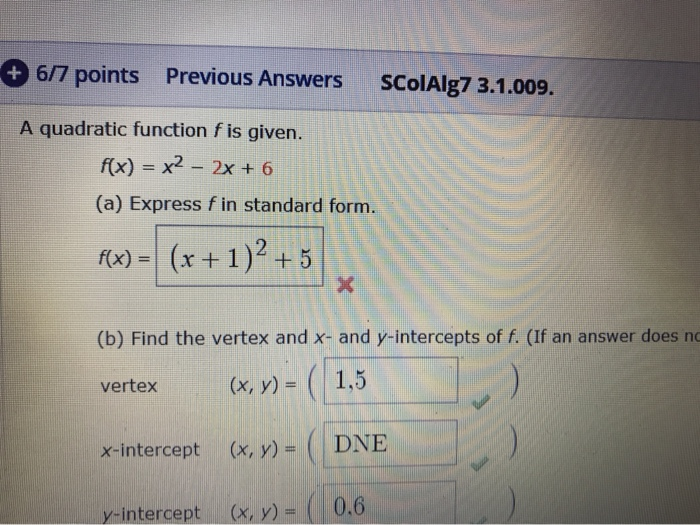+ 6/7 points Previous Answers ColAlg7 3.1.009. A quadratic function f is given. f(x) = x2 - 2x + 6 (a) Express fin standard form. f(x) = (x + 1)2 + 5 (b) Find the vertex and x- and y-intercepts of f. (If an answer does no vertex (x, y) = ( 1,5 x-intercept (x, y) = ( DNE y-intercept (x, y) = 0.6

• ### 3. List the vertex form of the quadratic function and state all relevant information. 4. Convert ...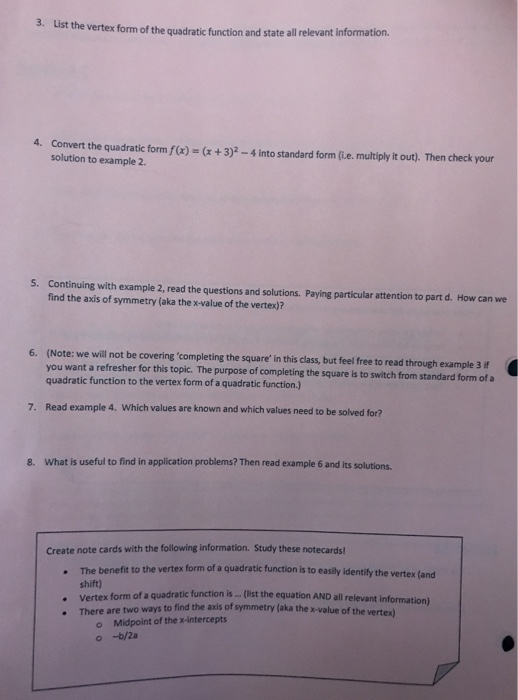3. List the vertex form of the quadratic function and state all relevant information. 4. Convert the quadratic form f(x) +3-4 into standard form (le. multiply t out). Then check your solution to example 2. 5. Continuing with example 2, read the questions and solutions. Paying particular attention to part d. How can we find the axis of symmetry (aka the x-value of the vertex)? 6. (Note: we will not be covering 'completing the square' in this class, but feel...

• ### 13. The following quadratic function is written in general form: f(x)=x2-6x-7 a) Write the factored form...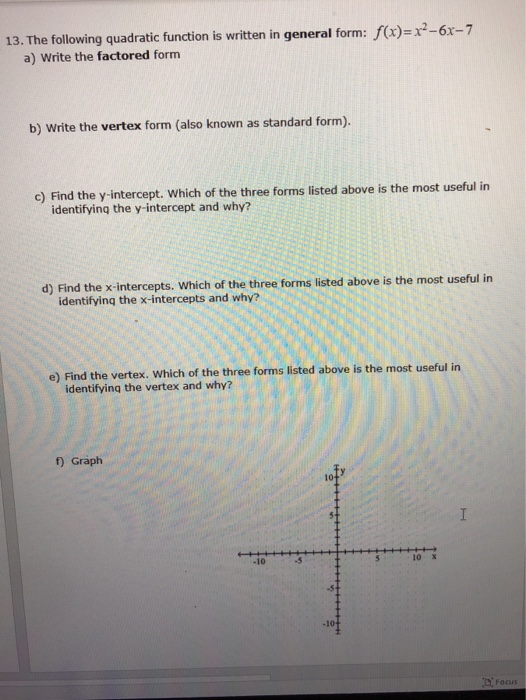13. The following quadratic function is written in general form: f(x)=x2-6x-7 a) Write the factored form b) Write the vertex form (also known as standard form). c) Find the y-intercept. Which of the three forms listed above is the most useful in identifying the y-intercept and why? d) Find the x-intercepts. Which of the three forms listed above is the most useful in identifying the x-intercepts and why? e) Find the vertex. Which of the three forms listed above is...

• ### Use the method of completing the square to find the standard form of the quadratic function....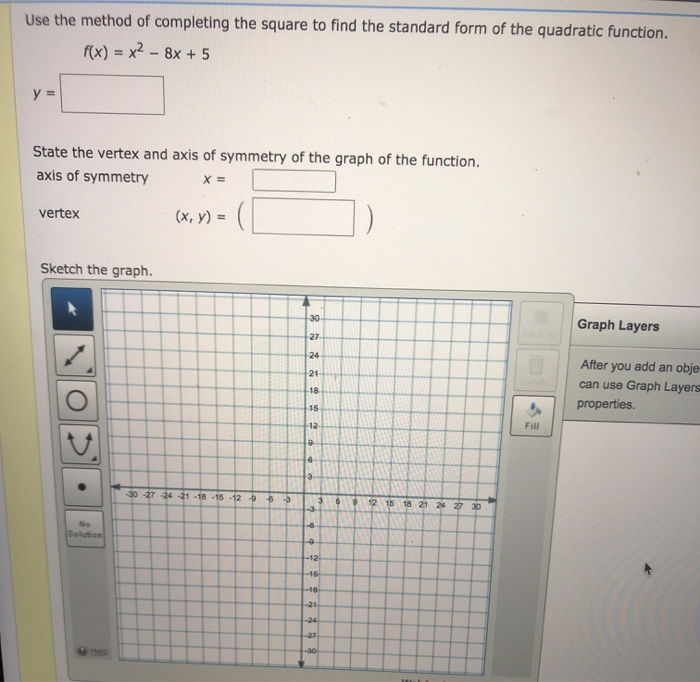Use the method of completing the square to find the standard form of the quadratic function. f(x) = x2 - 8x + 5 y = State the vertex and axis of symmetry of the graph of the function. axis of symmetry X = vertex (x, y) = Sketch the graph. 30 Graph Layers 27 24 21 After you add an obje can use Graph Layers properties. 18 15 -12 Fill 19 6 3 -30 -27 -24 -21 -18 -15 -12...

• ### A quadratic function fis given. F(x) = 1 - 4x - x² (a) Express fin standard...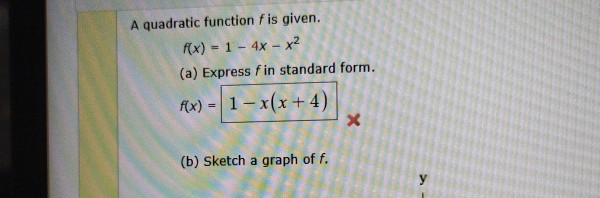A quadratic function fis given. F(x) = 1 - 4x - x² (a) Express fin standard form. f(x) - 1 - x(x +4) X (b) Sketch a graph of f. у

• ### Write a quadratic function in intercept form whose graph has the given x-intercepts and passes through the given point

1. Write a quadratic function in intercept form whose graph has the given x-intercepts and passes through the given point.x-intercepts: 2,3point: (4,2)2. Write a quadratic function in standard form whose graph passes through the given points.(1,-2),(-2,1),(3,6)

• ### Convert each quadratic function into vertex form

Convert each quadratic function into vertex form. State the vertex, axis of symmetry, and direction of opening: 1. y = 2x^2-4x+3